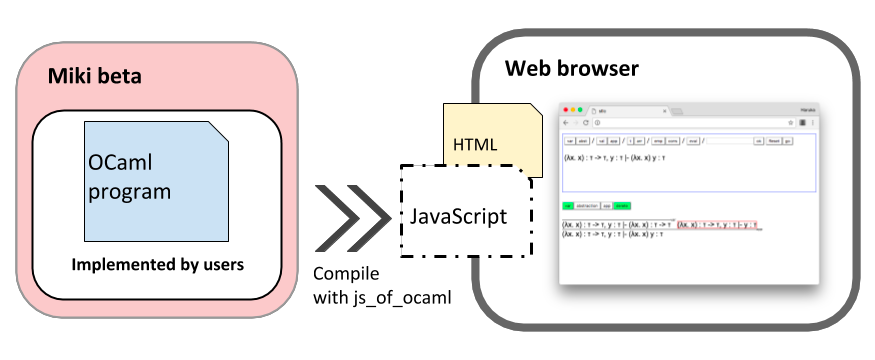# Miki beta

### What is Miki beta?

Miki Beta is a library to generate GUI for proof trees in any proof system.Proof trees are very helpful. They help visualize recursive procedures and allow us to understand how a conclusion can be derived from given premises. However, proof trees often grow bigger than your physical paper/whiteboard unless you carefully plan how you will use your space.
Miki Beta provides you with a GUI for visualizing proof trees. You can get the handy GUI easily and quickly by writing a bit of OCaml code that describes your proof system. Once you've got the GUI, you are able to grow proof trees by clicking. Even if the tree gets bigger than expected, you can scroll and resize it. You can also cut nodes without any eraser.

### Demo

You can play from here.

### OverviewTo construct a GUI for your proof system, you should give a single file, which defines the following three things.
1. Representation of types for each term/formula
2. How you want to draw each term/formula on screen
3. List of axioms and inference rules
See user-manual for details. The file is compiled together with Miki Beta, and converted into JavaScript. All programs are put together in a single JavaScript file. Now you get a GUI for your proof system on your web browser.

### Key technique - datatype-generic programming

Miki Beta is based on datatype-generic programming . Datatype-generic programming provides the genericity over the stucture of types. It realizes such a genericity by representation of datatypes with a sum-of-product structure. Here we will learn PolyP  as an example.
Two questions:
1. Define tree and list structures.
2. Implement a function `get_params` that returns a list of all values the given term (tree or list typed) contains
First, let us see how they can be defined in the standard OCaml.
(* Standard definition of tree datatype in OCaml *)
 type tree_t = | Leaf | Node of tree_t * int * tree_t

(* Standard definition of list datatype in OCaml *)
 type list_t = | Empty | Cons of int * list_t
In the above program, tree and list structures are defined as OCaml standard datatypes. Then, let's implement a `get_param` function for their types.
```(* get_params_for_tree: tree_t -> int list *)
let rec get_params_for_tree t = match t with
| Leaf -> []
| Node (l, n, r) -> (get_params_for_tree l) @ [n] @ (get_params_for_tree r)

(* get_params_for_list: list_t -> int list *)
let rec get_params_for_list t = match t with
| Empty -> []
| Cons (n, r) -> n :: (get_params_for_list r)```
Although the above two functions look similar to each other, you have to define them separately. It is because of the type dependency. Whenever you define a new datatype, you have to add a corresponding function.

On the other hand, using datatype-generic terms, the main functionality of `get_params` can be defined in a single function as follows.
(* Definition of the datatype to represent generic world *)
 type code_t = | U (* unit type *) | P (* parameter type *) | I (* recursive type *) | Plus of code_t * code_t (* sum type *) | Times of code_t * code_t (* product type *)

(* Definition of a represented tree datatype *)
let tree_type =
Plus (U, Times (Times (I, P), I))

(* Definition of a represented list datatype *)
let list_type =
Plus (U, Times (P, I))
```(* get_params: code_t -> code_t -> type_f -> int list *)
let rec get_params c r t = match (c, t) with
| (U, Unit ()) -> []
| (I, Rec (t')) -> get_params r r t'
| (P, Par (n)) -> [n]
| (Plus (c1, c2), Sum (Left (t'))) -> get_params c1 r t'
| (Plus (c1, c2), Sum (Right (t'))) -> get_params c2 r t'
| (Times (c1, c2), Pair (t1', t2')) -> (get_params c1 r t1') @ (get_params c2 r t2')
| _ -> failwith "Should not happen"

(* get_params_for_tree: type_f -> int list *)
let get_params_for_tree = get_params tree_type tree_type

(* get_params_for_list: type_f -> int list *)
let get_params_for_list = get_params list_type list_type
```
Even if you add another representation of datatype, the above function `get_params` works for it. This is how the datatype-generic programming makes your program smaller.

Miki Beta mixes features of PolyP and Indexed Functors . While the above example follows PolyP, Indexed Functor represents indexed datatypes so that it can construct mutually recursive datatypes.

### User manual

See the user-manual page.

### References

1. A Formal Comparison of Approaches to Datatype-Generic Programming - José Pedro Magalhães and Andres Löh
2. PolyP - a polytypic programming language extension - Patrik Jansson and Johan Jeuring
3. Generic programming with indexed functors - Andres Löh and José Pedro Magal